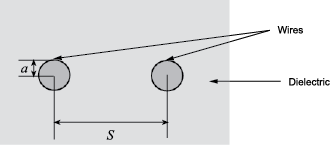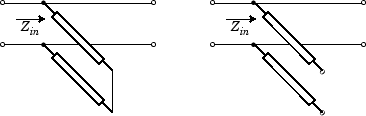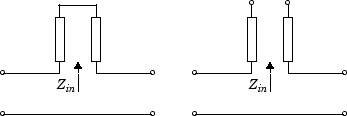# rfckt.twowire

Two-wire transmission line

## Description

Use the `rfckt.twowire` object to create two-wire transmission lines that are characterized by line dimensions, stub type, and termination.

A two-wire transmission line is shown in cross-section in the following figure. Its physical characteristics include the radius of the wires a, the separation or physical distance between the wire centers S, and the relative permittivity and permeability of the wires. RF Toolbox™ software assumes the relative permittivity and permeability are uniform.Note

`txlineTwoWire` is recommended over `rfckt.twowire` because it enables you to:

(since R2023b)

## Creation

### Syntax

``h = rfckt.twowire``
``h = rfckt.twowire(Name,Value)``

### Description

example

````h = rfckt.twowire` returns a shunt RLC network object whose properties all have their default values. The default object is equivalent to a pass-through 2-port network; i.e., the resistor, inductor, and capacitor are each replaced by a short circuit.```
````h = rfckt.twowire(Name,Value)` sets properties using one or more name-value pairs. For example, `rfckt.twowire('Radius',7.5e-4)` creates a two-wire transmission line with conducting wire radius of 7.5e-4 meters. You can specify multiple name-value pairs. Enclose each property name in a quote. Properties not specified retain their default values.```

## Properties

expand all

Computed S-parameters, noise figure, OIP3, and group delay values, specified as a `rfdata.data` object. This is a read-only property. For more information refer, Algorithms.

Data Types: `function_handle`

The separation or physical distance between the wire centers, specified as a scalar in meters. The default value is `0.0016`.

Data Types: `double`

Relative permittivity of dielectric, specified as a scalar. The relative permittivity is the ratio of permittivity of the dielectric,$\epsilon$, to the permittivity in free space, ${\epsilon }_{0}$. The default value is `2.3`.

Data Types: `double`

Physical length of transmission line, specified as a scalar in meters. The default value is `0.01`.

Data Types: `double`

Tangent of loss angle of dielectric, specified as a scalar. The default value is `0`.

Data Types: `double`

Relative permeability of dielectric, specified as a scalar. The ratio of permeability of dielectric, $\mu$, to the permeability in free space,

`${\mu }_{0}$`

. The default value is `1`.

Data Types: `double`

Object name, specified as a `1-by-N` character array. This is a read-only property.

Data Types: `char`

Number of ports, specified as a positive integer. This is a read-only property. The default value is `2`.

Data Types: `double`

Conducting wire radius, specified as a scalar in meters. The default value is `6.7e-4`.

Data Types: `double`

Conductor conductivity, specified as a scalar in Siemens per meter (S/m). The default value is `Inf`.

Data Types: `double`

Type of stub, specified as a one of the following values: `'NotaStub'`, `'Series'`, `'Shunt'`.

Data Types: `double`

Stub transmission line termination, specified as one of the following values: `'NotApplicable'`, `'Open'`, `'Short'`.

Data Types: `double`

## Object Functions

 `analyze` Analyze RFCKT object in frequency domain `calculate` Calculate specified parameters for rfckt objects or rfdata objects `circle` Draw circles on Smith Chart `extract` Extract specified network parameters from rfckt object or data object `listformat` List valid formats for specified circuit object parameter `listparam` List valid parameters for specified circuit object `loglog` Plot specified circuit object parameters using log-log scale `plot` Plot circuit object parameters on X-Y plane `plotyy` Plot parameters of RF circuit or RF data on X-Y plane with two Y-axes `getop` Display operating conditions `polar` Plot specified object parameters on polar coordinates `semilogx` Plot RF circuit object parameters using log scale for x-axis `semilogy` Plot RF circuit object parameters using log scale for y-axis `smith` Plot circuit object parameters on Smith chart `write` Write RF data from circuit or data object to file `getz0` Calculate characteristic impedance of RFCKT transmission line object `read` Read RF data from file to new or existing circuit or data object `restore` Restore data to original frequencies `getop` Display operating conditions `groupdelay` Group delay of S-parameter object or RF filter object or RF Toolbox circuit object

## Examples

collapse all

Create a two-wire transmission line object using `rfckt.twowire`.

`tx1=rfckt.twowire('Radius',7.5e-4)`
```tx1 = rfckt.twowire with properties: Radius: 7.5000e-04 Separation: 0.0016 MuR: 1 EpsilonR: 2.3000 LossTangent: 0 SigmaCond: Inf LineLength: 0.0100 StubMode: 'NotAStub' Termination: 'NotApplicable' nPort: 2 AnalyzedResult: [] Name: 'Two-Wire Transmission Line' ```

## Algorithms

• If you model the transmission line as a stubless line, the `analyze` method first calculates the ABCD-parameters at each frequency contained in the modeling frequencies vector. It then uses the `abcd2s` function to convert the ABCD-parameters to S-parameters.

The `analyze` method calculates the ABCD-parameters using the physical length of the transmission line, d, and the complex propagation constant, k, using the following equations:

`$\begin{array}{l}A=\frac{{e}^{kd}+{e}^{-kd}}{2}\\ B=\frac{{Z}_{0}*\left({e}^{kd}-{e}^{-kd}\right)}{2}\\ C=\frac{{e}^{kd}-{e}^{-kd}}{2*{Z}_{0}}\\ D=\frac{{e}^{kd}+{e}^{-kd}}{2}\end{array}$`

Z0 and k are vectors whose elements correspond to the elements of f, the vector of frequencies specified in the `analyze` input argument `freq`. Both can be expressed in terms of the resistance (R), inductance (L), conductance (G), and capacitance (C) per unit length (meters) as follows:

`$\begin{array}{c}{Z}_{0}=\sqrt{\frac{R+j2\pi fL}{G+j2\pi fC}}\\ k={k}_{r}+j{k}_{i}=\sqrt{\left(R+j2\pi fL\right)\left(G+j2\pi FC\right)}\end{array}$`

where

`$\begin{array}{l}R=\frac{1}{\pi a{\sigma }_{cond}{\delta }_{cond}}\\ L=\frac{\mu }{\pi }\text{a}\mathrm{cosh}\left(\frac{D}{2a}\right)\\ G=\frac{\pi \omega {\epsilon }^{″}}{\text{a}\mathrm{cosh}\left(\frac{D}{2a}\right)}\\ C=\frac{\pi \epsilon }{\text{a}\mathrm{cosh}\left(\frac{D}{2a}\right)}\end{array}$`

In these equations:

• w is the plate width.

• d is the plate separation.

• σcond is the conductivity in the conductor.

• μ is the permeability of the dielectric.

• ε is the permittivity of the dielectric.

• ε″ is the imaginary part of ε, ε″  = ε0εrtan δ, where:

• ε0 is the permittivity of free space.

• εr is the `EpsilonR` property value.

• tan δ is the `LossTangent` property value.

• δcond is the skin depth of the conductor, which the block calculates as $1/\sqrt{\pi f\mu {\sigma }_{cond}}$.

• f is a vector of modeling frequencies determined by the Outport (RF Blockset) block.

• If you model the transmission line as a shunt or series stub, the `analyze` method first calculates the ABCD-parameters at the specified frequencies. It then uses the `abcd2s` function to convert the ABCD-parameters to S-parameters.

When you set the `StubMode` property to `'Shunt'`, the 2-port network consists of a stub transmission line that you can terminate with either a short circuit or an open circuit as shown in the following figure.Zin is the input impedance of the shunt circuit. The ABCD-parameters for the shunt stub are calculated as:

`$\begin{array}{c}A=1\\ B=0\\ C=1/{Z}_{in}\\ D=1\end{array}$`

When you set the `StubMode` property to `'Series'`, the 2-port network consists of a series transmission line that you can terminate with either a short circuit or an open circuit as shown in the following figure.Zin is the input impedance of the series circuit. The ABCD-parameters for the series stub are calculated as:

`$\begin{array}{c}A=1\\ B={Z}_{in}\\ C=0\\ D=1\end{array}$`

 Pozar, David M. Microwave Engineering, John Wiley & Sons, Inc., 2005.